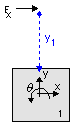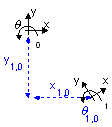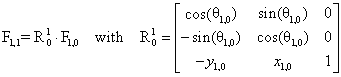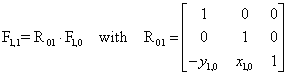﻿ 20-sim webhelp > Library > Iconic Diagrams > Mechanical > Translation > 2DSmallRotation > Small Rotations

# Small Rotations

Navigation:  Library > Iconic Diagrams > Mechanical > Translation > 2DSmallRotation >

# Small RotationsConsider the body below:Given a force Fx in x-direction acting upon the body at an offset y1 from the center, the resulting force at the center of the body is:

F1x = Fx * cos(q)

F1y = Fx * sin(q)

F1q = Fx * -y1

For small angles this can be simplified to:

F1x = Fx

F1y = 0

F1q = Fx * -y1

A force acting upon a body at a certain offset is rewritten to a force at the center of the body. This is common in multibody dynamics and called a transformation. A force acting at a certain point can be transformed to see its effect at another point.

Consider two frames which have an arbitrary offset as shown in the picture below. We want to know how a force in frame 0 is transformed to a force in frame 1. We assume that frame 1 has an offset of x1 and y1 with respect to frame 0 and a rotation q1 with respect to frame 0. In order to make clear that the offsets are given with respect to frame 0 we will write the with extra indices (x1,0 , y1,0 and q1,0 ).Given an arbitrary force F1,0 at frame 0 the resulting force F1,1 at frame 1 is equal to:For small angles this can be simplified to:The accuracy of this simplification depends on the angle q. For an angle of 0.5 degrees (0.009 rad) this results in an almost 1% error in the calculation of F1,1. Considering the cumulative effects of this error when multiple models are used, an angle of maximum 0.05 degrees (<1 mrad) is an upper bound for the models of the 2DSmallRotation library.

The simplification of the equations has two advantages. First because of the small rotations there is no frame rotation any more. Therefore all frames have the same direction (there is only an offset in x-direction and y-direction). Interpreting results is therefore much easier. But the biggest advantage is the simplification of equations which allows us to linearize models symbolically and thus perform tasks in the frequency like parameter sweeps!